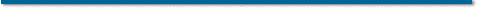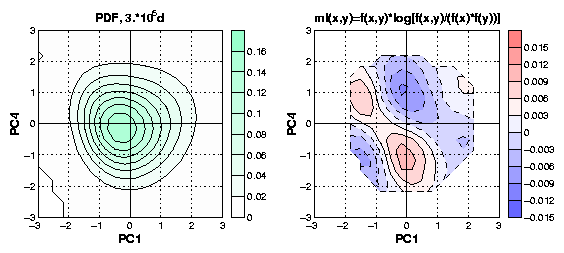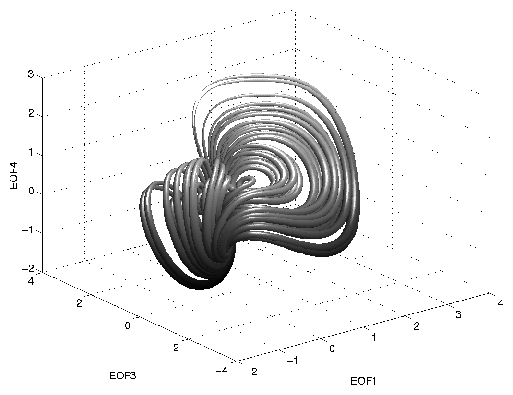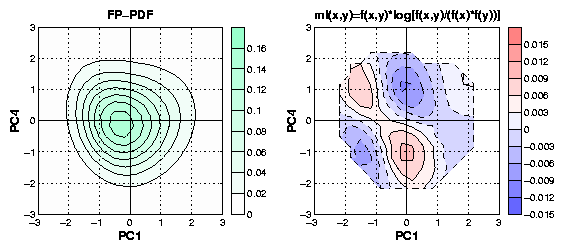# A nonlinear stochastic model of atmospheric low-frequency behavior variability

Judith Berner
University of Bonn, GermanyAbstract

Although there is agreement that the atmosphere is fundamentally nonlinear, much of low-frequency behavior has been interpreted in terms of a linear system. A thorough analysis of nonlinear signatures is often hampered by the data requirements for such a study. With this issue in mind, an exceptionally long atmospheric general circulation model (GCM) integration of NCAR's CCM0 is studied to examine the influence of nonlinearities on low-frequency behavior.

Two approaches reveal nonlinear signatures in projections of the 500-hPa height field onto phase space planes defined by the leading empirical orthogonal functions (EOFs):

1. The probability density function (PDF) exhibits departures from a multivariate normal function, though no more than one maximum is found (Fig.1).Fig.1: a) PDF of the geopotential height in the 2D phase space spanned by EOF1 and EOF3. b) The mutual information density f(x,y)*log[f(x,y)/(f(x)*f(y))] emphasizing the density "riges" (with regard to a bivarite Gaussian) of the PDF in a).
2. In some planes the mean phase space velocities (or mean drift) together with the trajectories they imply produce distinct signatures of more than one equilibrium point (Fig.2).Fig.2: Mean trajectory in the 3D-EOF phase space spanned by EOF1, EOF3 and EOF4. The nonlinear tendencies are calculated by removing the damping component of a least mean square linear fit from the mean tendencies.
With this in mind low-dimensional stochastic models that approximate the behavior of the GCM in EOF-phase space are built. It turns out that there is not a unique solution, but that there exists a class of "stochastically equivalent" models with the same drift and diffusion as the GCM. Two models in this class are studied in more detail: one with a linear deterministic part and multiplicative noise and one with a nonlinear deterministic part and additive noise. The stationary PDF of these models agrees remarkably well with the GCM's PDF. Moreover, we show to what degree even the temporal behavior is captured by the stochastic models.

We argue that of all low-dimensional stochastic models the most simple is the model with nonlinear deterministic part and additive white noise (Fig.3). This model represents the stationary and the temporal behavior of the GCM geopotential heights in a remarkable mannerFig.3: a) PDF of of a 2D nonlinear stochastic model, with nonlinear deterministic part derived from the GCM dataset. b) The mutual information density PDF(x,y)/(PDF(x)*PDF(y)) emphasizing the density "riges" (with regard to a bivarite Gaussian) of the PDF in a).30 Oct, 2001
2 PM/ DSRC 1D 403
(Coffee at 1:50 PM)
Back to PSL seminar list.